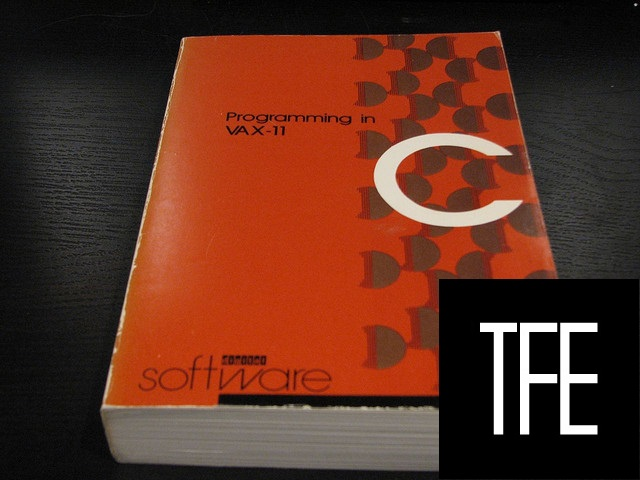# What is “:-!!” in C code?I bumped into this strange macro code in /usr/include/linux/kernel.h:

``````/* Force a compilation error if condition is true, but also produce a
result (of value 0 and type size_t), so the expression can be used
e.g. in a structure initializer (or where-ever else comma expressions
aren't permitted). */
#define BUILD_BUG_ON_ZERO(e) (sizeof(struct { int:-!!(e); }))
#define BUILD_BUG_ON_NULL(e) ((void *)sizeof(struct { int:-!!(e); }))``````

What does `:-!!` do?

chmurli

This is, in effect, a way to check whether the expression e can be evaluated to be 0, and if not, to fail the build.

The macro is somewhat misnamed; it should be something more like `BUILD_BUG_OR_ZERO`, rather than`...ON_ZERO`. (There have been occasional discussions about whether this is a confusing name.)

You should read the expression like this:

``sizeof(struct { int: -!!(e); }))``
1. `(e)`: Compute expression `e`.
2. `!!(e)`: Logically negate twice: `0` if `e == 0`; otherwise `1`.
3. `-!!(e)`: Numerically negate: `0` if `e == 0`; otherwise `-1`.
4. `struct{int: -!!(0);} --> struct{int: 0;}`: If it was zero, then we declare a struct with an anonymous integer bitfield that has width zero. Everything is fine and we proceed as normal.
5. `struct{int: -!!(1);} --> struct{int: -1;}`: On the other hand, if it isn’t zero, then it will be some negative number. Declaring any bitfield with negative width is a compilation error.

So we’ll either wind up with a bitfield that has width 0 in a struct, which is fine, or a bitfield with negative width, which is a compilation error. Then we take `sizeof` that field, so we get a `size_t` with the appropriate width (which will be zero in the case where `e` is zero).

Some people have asked: Why not just use an `assert`?

keithmo’s answer here has a good response:

These macros implement a compile-time test, while assert() is a run-time test.

Exactly right. You don’t want to detect problems in your kernel at runtime that could have been caught earlier! It’s a critical piece of the operating system. To whatever extent problems can be detected at compile time, so much the better.

John Feminella

Stack Overflow